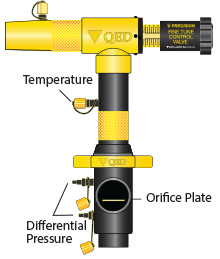# Orifice Plate Flow CalculatorTemp (F)
CH4/CO2 Ratio (60/40) or AIR
Delta P (inch-H2O)
Applied Vac (inch-H2O)
Pipe Size
Plate Size (inch) + (pipe)
LFG (bal=AIR) Flow (cfm)
23.5

QED orifice flow calculation tool uses AGA and ASME orifice flow calculation methods to provide an estimate of gas flows given a limited set of input data (gas temperature, CH4/CO2 ratio, applied vacuum, QED orifice size, pipe size and resulting delta-Pressure across the orifice). Assumptions include saturated gas and CH4+CO2 add to 100%. If CH4 + CO2 are less than 100%, then it uses AIR as the balance gas (and the label reads -- LFG (bal=AIR) Flow (cfm)) If CH4 + CO2 are greater than 100%, then it uses the ratio of CH4/CO2 to adjust the values to 100% (LFG (ratio fix) Flow (cfm)). Values were derived using an Industrial Flow Calculator for LFG at 100oF, 50/50 mix of CH4 and CO2, with 20 inches of applied vacuum at 100% humidity.

Lab testing has shown excellent agreement between lab measured air flows and tool results. Tool results may not always be accurate, depending on the accuracy of field data. Check tool results against readings from other methods

close